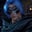Related Tags

communitycreator
python

# How to calculate a cumulative sum in PythonGutha Vamsi Krishna

In this shot, we will learn how to calculate a cumulative sum in Python.

The cumulative sum is the sum of given numbers as it grows with the sequence. We will start by defining the problem statement, followed by an example.

### Problem statement

Given a list of numbers, ln, calculate the cumulative sum for the list.

### Example

Input: ln = [1, 2, 3, 4, 5, 6, 7, 8, 9, 10]

Output: [1, 3, 6, 10, 15, 21, 28, 36, 45, 55]

#### Explanation

• 1 ===> 1

• 3 ===> 1 + 2

• 6 ===> 1 + 2 + 3

• 55 ===> 1 + 2 + 3 + 4 + 5 + 6 + 7 + 8 + 9 + 10

### Implementation

#given list
ln = [1, 2, 3, 4, 5, 6, 7, 8, 9, 10]

#create empty cumulative list
cl = []

#declare variable for sum
s = 0

#use for loop and append s to cl
for i in ln:
s += i
cl.append(s)

#print cumulative list
print(cl)
Calculating cumulative sum in Python

### Explanation

• Line 2: We get the given list of numbers, ln.

• Line 5: We declare an empty list cl to store the cumulative sum.

• Line 8: We declare and initialize variable s, which keeps track of the sum of previous numbers.

• Line 11: We use a for loop to traverse the given list ln.

• Line 12: We add the present number i to sum s.

• Line 13: We append the sum s to cumulative list cl.

• Line 16: We print cl, which contains the cumulative sum for the given list of numbers.

RELATED TAGS

communitycreator
python

CONTRIBUTORGutha Vamsi Krishna
RELATED COURSES

View all Courses

Keep Exploring

Learn in-demand tech skills in half the time